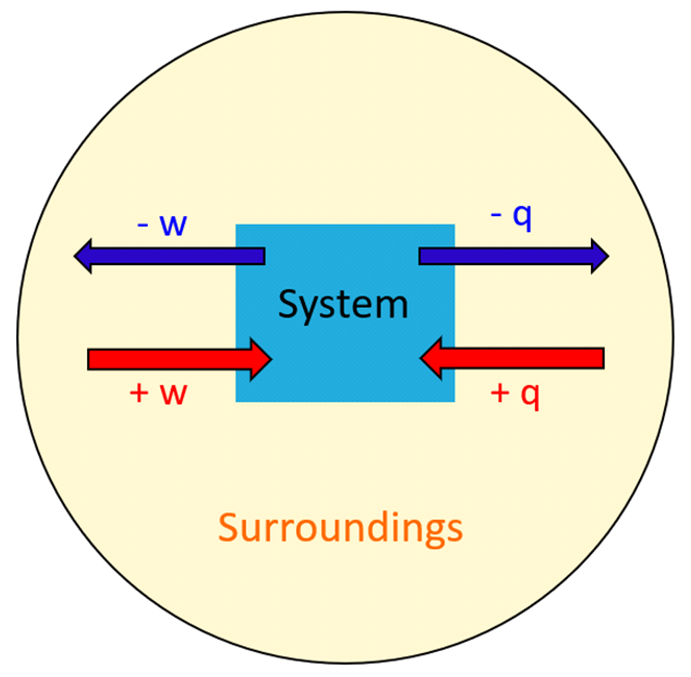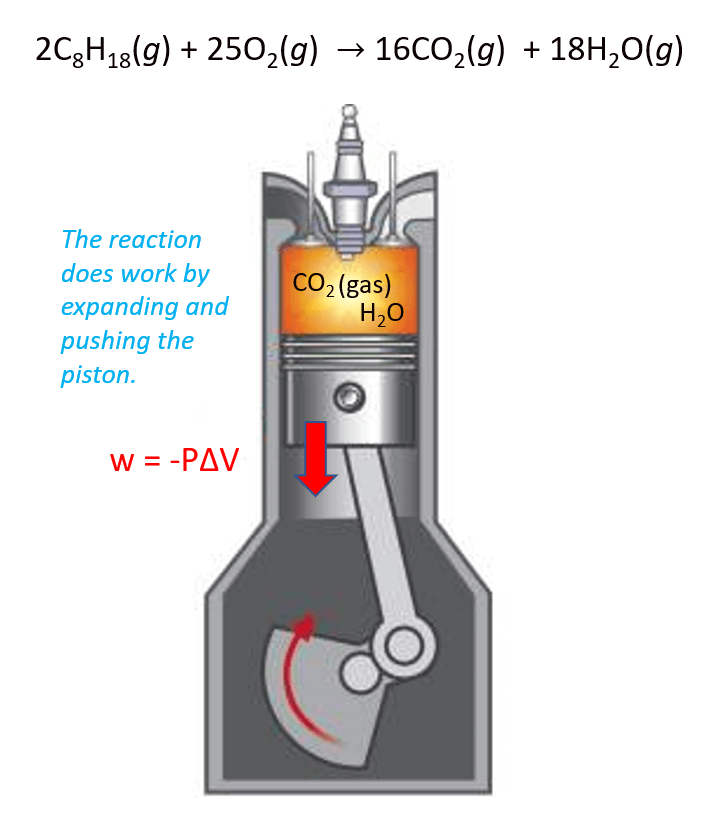## General Chemistry

The first law of thermodynamics is the law of energy conservation, which states that energy can be converted from one form to another, but cannot be created or destroyed.

One implication of this law is that the total energy of the universe is constant. The energy transfers from a system to its surroundings but it is never lost.

The system is what we are interested in, while everything else is the surroundings.

The next question is how does the energy transfer take place? There are two ways the energy transfers between the system and surroundings; heat (q) and work (w).So, energy is the capability of doing work or producing heat. Anytime the system does work or gives off heat, its energy decreases, and therefore, the q and w have negative signs. On the contrary, when work is done on the system or it absorbs heat, its internal energy increases (+q, +w).Think of the internal energy as a bank account, it increases when work is done on it, and it decreases if a work is done by it.

For example,

A gas absorbs 62 kJ of heat and does 48 kJ of work. Calculate ΔE.

The internal energy change is equal to:

Δ= q + w

If the heat is absorbed by the system, then it has a positive sign. The job done by the system indicates a negative sign for w.

Δ= +62 kJ – 48 kJ = 16 kJ

# Work and Energy

Work can be defined as force F multiplied by distance d: w = F x d

Although work has a very broad definition covering many aspects of thermodynamics, in this section, we study the work done by a gas that expands by moving the weightless cylinder of a piston.

Perhaps the best example here is the combustion of gasoline. The products CO2 and H2O gases expand the volume and thus move the piston which is the work done by the reaction.In this case, the work is calculated by the following formula:

w = –PΔV

where P is the pressure exerted by the gas, and ΔV is its volume change.

Example:

A pump is held under 1.30 atm external pressure. How much work (in J) is required to expand its volume from 1.80 L to 3.50 L?

W = -PΔV

W = -1.30 atm (3.50 L – 1.80 L) = -2.21 atm · L

The last step is to convert atm · L to Joules. 1 L· atm = 101.3 J, therefore:

${\rm{w}}\;{\rm{ = }}\;{\rm{ – 2}}{\rm{.21 }}\cancel{{{\rm{atm}}\;{\rm{\cdot}}\;{\rm{L}}}}\;{\rm{ \times }}\;\frac{{{\rm{101}}{\rm{.3 J}}}}{{{\rm{1}}\;\cancel{{{\rm{atm}}\;{\rm{\cdot}}\;{\rm{L}}}}}}\;{\rm{ = }}\;{\rm{224}}\;{\rm{J}}\;$

# Energy Change in Chemical Reactions

In chemistry, the system is the reaction and all the changes in energy are always looked at from the perspective of the system/reaction.

For example, the system can be a flask where the reaction takes place, or it can just be the reaction while the solvent in which the reactants and products are dissolved is already part of the surroundings.

A chemical reaction that gives off heat to its surroundings is called an exothermic reaction (negative q).

Endothermic reactions (endo means “within,” so heat flows in), on the other hand, absorb heat from surroundings, and q, in this case, is positive.ΔH is the enthalpy change of the reaction and will cover it separately, however, for now, take it as if it is the heat since most often it is.

The energy change of chemical reactions can be calculated using calorimetry, and heats for formations by a direct method or using the Hess’s law. The links to the corresponding topics are shown below.

#### Practice

1.

What is the ΔE of the system which does 42.0 kJ of work on the surroundings and absorbs 51.0 kJ of heat?

This content is available to registered users only. Click here to Register! By joining Chemistry Steps, you will gain instant access to the answers and solutions for all the Practice Problems and the powerful set of General Chemistry 1 and 2 Summary Study Guides.
Solution
This content is available to registered users only. Click here to Register! By joining Chemistry Steps, you will gain instant access to the answers and solutions for all the Practice Problems and the powerful set of General Chemistry 1 and 2 Summary Study Guides.
2.

Calculate Δfor each of the following.

a) = -62 kJ, w = +59 kJ

b) = +48 kJ, w = –56 kJ

c) = -92 kJ, w 0 kJ

This content is available to registered users only. Click here to Register! By joining Chemistry Steps, you will gain instant access to the answers and solutions for all the Practice Problems and the powerful set of General Chemistry 1 and 2 Summary Study Guides.
Solution
This content is available to registered users only. Click here to Register! By joining Chemistry Steps, you will gain instant access to the answers and solutions for all the Practice Problems and the powerful set of General Chemistry 1 and 2 Summary Study Guides.
3.

The gas in a piston is heated up by absorbing 984 J of heat and expands performing 541 J of work on the surroundings.

What is the change in internal energy for the system (the gas)?

This content is available to registered users only. Click here to Register! By joining Chemistry Steps, you will gain instant access to the answers and solutions for all the Practice Problems and the powerful set of General Chemistry 1 and 2 Summary Study Guides.
Solution
This content is available to registered users only. Click here to Register! By joining Chemistry Steps, you will gain instant access to the answers and solutions for all the Practice Problems and the powerful set of General Chemistry 1 and 2 Summary Study Guides.
4.

Calculate the change in internal energy of the system if it releases 415 kJ of heat and does 562 kJ of work on the surroundings.